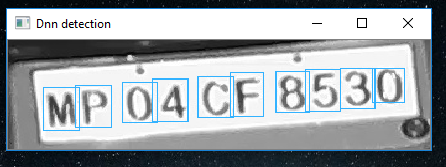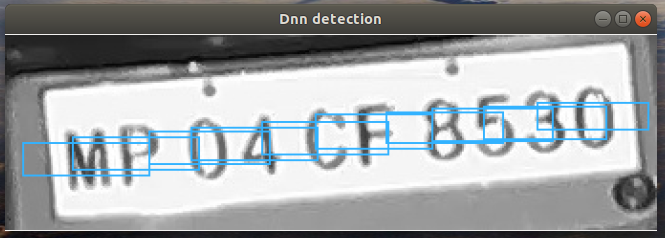# Revision history [back]

### System information (version)

• OpenCV => 4.0.1
• Operating System / Platform => Windows 10 64 Bit
• Compiler => Visual Studio 2015

• Operating System / Platform => Ubuntu 18.04.1 LTS 64 Bit

• Compiler => gcc 7.4.0

### Detailed description

I have a custom network based on tiny yolov2 and it is trained using the darknet framework. Now when i do inference using this network on windows the results are as expected, But on ubuntu results are very different and not as expected. - on windows• on ubuntuI have tried different version of opencv (4.01, 3.4.4, 3.4.2) and on windows the results are always correct but on ubuntu thery are always wrong.

Also i have tried darknet's C++ API and results in it are correct on both windows and ubuntu but i cannot use it because its CPU inference is very slow compared to opencv's.

Also it might look as if only the bbox's are wrong but sometimes the number of objects detected are are also less on ubuntu compared to windows.

The issue seems to be only for this network, i have tried tiny-yolov3, tiny-yolov2 and SSD on both windows and ubuntu and they work fine on both platforms.

### Steps to reproduce

model files and test images

#include <string>
#include <vector>
#include <opencv2/opencv.hpp>

using namespace cv;
using namespace dnn;
using namespace std;

float confThreshold = 0.5; // Confidence threshold
float nmsThreshold = 0.4;  // Non-maximum suppression threshold
int inpWidth = 288;  // Width of network's input image
int inpHeight = 128; // Height of network's input image
vector<string> classes;

void postprocess(Mat& frame, const vector<Mat>& out);
void drawPred(int classId, float conf, int left, int top, int right, int bottom, Mat& frame);
vector<String> getOutputsNames(const Net& net)

int main(int argc, char** argv) {

classes.push_back("char");

std::string image_file_path = "images/img_6.jpg";

net.setPreferableBackend(DNN_BACKEND_OPENCV);
net.setPreferableTarget(DNN_TARGET_CPU);

Mat blob;
blobFromImage(frame, blob, 1 / 255.0, cv::Size(inpWidth, inpHeight), Scalar(0, 0, 0), true, false);

vector<Mat> outs;
net.forward(outs, getOutputsNames(net));

postprocess(frame, outs);

imshow("detections", detection_img);
cv::waitKey(0);

}

vector<String> getOutputsNames(const Net& net)
{
static vector<String> names;
if (names.empty())
{
//Get the indices of the output layers, i.e. the layers with unconnected outputs
vector<int> outLayers = net.getUnconnectedOutLayers();

//get the names of all the layers in the network
vector<String> layersNames = net.getLayerNames();

// Get the names of the output layers in names
names.resize(outLayers.size());
for (size_t i = 0; i < outLayers.size(); ++i)
names[i] = layersNames[outLayers[i] - 1];
}
return names;
}

void postprocess(Mat& frame, const vector<Mat>& outs)
{
vector<int> classIds;
vector<float> confidences;
vector<Rect> boxes;

for (size_t i = 0; i < outs.size(); ++i)
{
// Scan through all the bounding boxes output from the network and keep only the
// ones with high confidence scores. Assign the box's class label as the class
// with the highest score for the box.
float* data = (float*)outs[i].data;
for (int j = 0; j < outs[i].rows; ++j, data += outs[i].cols)
{
Mat scores = outs[i].row(j).colRange(5, outs[i].cols);
Point classIdPoint;
double confidence;
// Get the value and location of the maximum score
minMaxLoc(scores, 0, &confidence, 0, &classIdPoint);
if (confidence > confThreshold)
{
int centerX = (int)(data * frame.cols);
int centerY = (int)(data * frame.rows);
int width = (int)(data * frame.cols);
int height = (int)(data * frame.rows);
int left = centerX - width / 2;
int top = centerY - height / 2;

classIds.push_back(classIdPoint.x);
confidences.push_back((float)confidence);
boxes.push_back(Rect(left, top, width, height));
}
}
}

// Perform non maximum suppression to eliminate redundant overlapping boxes with
// lower confidences
vector<int> indices;
NMSBoxes(boxes, confidences, confThreshold, nmsThreshold, indices);
for (size_t i = 0; i < indices.size(); ++i)
{
int idx = indices[i];
Rect box = boxes[idx];
rectangle(frame, Point(box.x, box.y), Point(box.x + box.width, box.y + box.height), Scalar(255, 178, 50), 1);
}
}


i have raised a opencv issue for this problem here

### System information (version)

• OpenCV => 4.0.1
• Operating System / Platform => Windows 10 64 Bit
• Compiler => Visual Studio 2015

• Operating System / Platform => Ubuntu 18.04.1 LTS 64 Bit

• Compiler => gcc 7.4.0

### Detailed description

I have a custom network based on tiny yolov2 and it is trained using the darknet framework. Now when i do inference using this network on windows the results are as expected, But on ubuntu results are very different and not as expected. - on windows• on ubuntuI have tried different version of opencv (4.01, 3.4.4, 3.4.2) and on windows the results are always correct but on ubuntu thery are always wrong.

Also i have tried darknet's C++ API and results in it are correct on both windows and ubuntu but i cannot use it because its CPU inference is very slow compared to opencv's.

Also it might look as if only the bbox's are wrong but sometimes the number of objects detected are are also less on ubuntu compared to windows.

The issue seems to be only for this network, i have tried tiny-yolov3, tiny-yolov2 and SSD on both windows and ubuntu and they work fine on both platforms.

### Steps to reproduce

model files and test images

#include <string>
#include <vector>
#include <opencv2/opencv.hpp>

using namespace cv;
using namespace dnn;
using namespace std;

float confThreshold = 0.5; // Confidence threshold
float nmsThreshold = 0.4;  // Non-maximum suppression threshold
int inpWidth = 288;  // Width of network's input image
int inpHeight = 128; // Height of network's input image
vector<string> classes;

void postprocess(Mat& frame, const vector<Mat>& out);
void drawPred(int classId, float conf, int left, int top, int right, int bottom, Mat& frame);
vector<String> getOutputsNames(const Net& net)

int main(int argc, char** argv) {

classes.push_back("char");

std::string image_file_path = "images/img_6.jpg";

net.setPreferableBackend(DNN_BACKEND_OPENCV);
net.setPreferableTarget(DNN_TARGET_CPU);

Mat blob;
blobFromImage(frame, blob, 1 / 255.0, cv::Size(inpWidth, inpHeight), Scalar(0, 0, 0), true, false);

vector<Mat> outs;
net.forward(outs, getOutputsNames(net));

postprocess(frame, outs);

imshow("detections", detection_img);
cv::waitKey(0);

}

vector<String> getOutputsNames(const Net& net)
{
static vector<String> names;
if (names.empty())
{
//Get the indices of the output layers, i.e. the layers with unconnected outputs
vector<int> outLayers = net.getUnconnectedOutLayers();

//get the names of all the layers in the network
vector<String> layersNames = net.getLayerNames();

// Get the names of the output layers in names
names.resize(outLayers.size());
for (size_t i = 0; i < outLayers.size(); ++i)
names[i] = layersNames[outLayers[i] - 1];
}
return names;
}

void postprocess(Mat& frame, const vector<Mat>& outs)
{
vector<int> classIds;
vector<float> confidences;
vector<Rect> boxes;

for (size_t i = 0; i < outs.size(); ++i)
{
// Scan through all the bounding boxes output from the network and keep only the
// ones with high confidence scores. Assign the box's class label as the class
// with the highest score for the box.
float* data = (float*)outs[i].data;
for (int j = 0; j < outs[i].rows; ++j, data += outs[i].cols)
{
Mat scores = outs[i].row(j).colRange(5, outs[i].cols);
Point classIdPoint;
double confidence;
// Get the value and location of the maximum score
minMaxLoc(scores, 0, &confidence, 0, &classIdPoint);
if (confidence > confThreshold)
{
int centerX = (int)(data * frame.cols);
int centerY = (int)(data * frame.rows);
int width = (int)(data * frame.cols);
int height = (int)(data * frame.rows);
int left = centerX - width / 2;
int top = centerY - height / 2;

classIds.push_back(classIdPoint.x);
confidences.push_back((float)confidence);
boxes.push_back(Rect(left, top, width, height));
}
}
}

// Perform non maximum suppression to eliminate redundant overlapping boxes with
// lower confidences
vector<int> indices;
NMSBoxes(boxes, confidences, confThreshold, nmsThreshold, indices);
for (size_t i = 0; i < indices.size(); ++i)
{
int idx = indices[i];
Rect box = boxes[idx];
rectangle(frame, Point(box.x, box.y), Point(box.x + box.width, box.y + box.height), Scalar(255, 178, 50), 1);
}
}


i have no idea where to start further debugging to solve this problem.

i have raised a opencv issue for this problem here3 Nonesupra56945 ●9 ●6

### System information (version)

• OpenCV => 4.0.1
• Operating System / Platform => Windows 10 64 Bit
• Compiler => Visual Studio 2015

• Operating System / Platform => Ubuntu 18.04.1 LTS 64 Bit

• Compiler => gcc 7.4.0

### Detailed description

I have a custom network based on tiny yolov2 and it is trained using the darknet framework. Now when i do inference using this network on windows the results are as expected, But on ubuntu results are very different and not as expected. - on windows• on ubuntuI have tried different version of opencv (4.01, 3.4.4, 3.4.2) and on windows the results are always correct but on ubuntu thery are always wrong.

Also i have tried darknet's C++ API and results in it are correct on both windows and ubuntu but i cannot use it because its CPU inference is very slow compared to opencv's.

Also it might look as if only the bbox's are wrong but sometimes the number of objects detected are are also less on ubuntu compared to windows.

The issue seems to be only for this network, i have tried tiny-yolov3, tiny-yolov2 and SSD on both windows and ubuntu and they work fine on both platforms.

### Steps to reproduce

model files and test images

#include <string>
#include <vector>
#include <opencv2/opencv.hpp>

using namespace cv;
using namespace dnn;
using namespace std;

float confThreshold = 0.5; // Confidence threshold
float nmsThreshold = 0.4;  // Non-maximum suppression threshold
int inpWidth = 288;  // Width of network's input image
int inpHeight = 128; // Height of network's input image
vector<string> classes;

void postprocess(Mat& frame, const vector<Mat>& out);
void drawPred(int classId, float conf, int left, int top, int right, int bottom, Mat& frame);
vector<String> getOutputsNames(const Net& net)

int main(int argc, char** argv) {

classes.push_back("char");

std::string image_file_path = "images/img_6.jpg";

net.setPreferableBackend(DNN_BACKEND_OPENCV);
net.setPreferableTarget(DNN_TARGET_CPU);

Mat blob;
blobFromImage(frame, blob, 1 / 255.0, cv::Size(inpWidth, inpHeight), Scalar(0, 0, 0), true, false);

vector<Mat> outs;
net.forward(outs, getOutputsNames(net));

postprocess(frame, outs);

imshow("detections", detection_img);
cv::waitKey(0);

}

vector<String> getOutputsNames(const Net& net)
{
static vector<String> names;
if (names.empty())
{
//Get the indices of the output layers, i.e. the layers with unconnected outputs
vector<int> outLayers = net.getUnconnectedOutLayers();

//get the names of all the layers in the network
vector<String> layersNames = net.getLayerNames();

// Get the names of the output layers in names
names.resize(outLayers.size());
for (size_t i = 0; i < outLayers.size(); ++i)
names[i] = layersNames[outLayers[i] - 1];
}
return names;
}

void postprocess(Mat& frame, const vector<Mat>& outs)
{
vector<int> classIds;
vector<float> confidences;
vector<Rect> boxes;

for (size_t i = 0; i < outs.size(); ++i)
{
// Scan through all the bounding boxes output from the network and keep only the
// ones with high confidence scores. Assign the box's class label as the class
// with the highest score for the box.
float* data = (float*)outs[i].data;
for (int j = 0; j < outs[i].rows; ++j, data += outs[i].cols)
{
Mat scores = outs[i].row(j).colRange(5, outs[i].cols);
Point classIdPoint;
double confidence;
// Get the value and location of the maximum score
minMaxLoc(scores, 0, &confidence, 0, &classIdPoint);
if (confidence > confThreshold)
{
int centerX = (int)(data * frame.cols);
int centerY = (int)(data * frame.rows);
int width = (int)(data * frame.cols);
int height = (int)(data * frame.rows);
int left = centerX - width / 2;
int top = centerY - height / 2;

classIds.push_back(classIdPoint.x);
confidences.push_back((float)confidence);
boxes.push_back(Rect(left, top, width, height));
}
}
}

// Perform non maximum suppression to eliminate redundant overlapping boxes with
// lower confidences
vector<int> indices;
NMSBoxes(boxes, confidences, confThreshold, nmsThreshold, indices);
for (size_t i = 0; i < indices.size(); ++i)
{
int idx = indices[i];
Rect box = boxes[idx];
rectangle(frame, Point(box.x, box.y), Point(box.x + box.width, box.y + box.height), Scalar(255, 178, 50), 1);
}
}


i have no idea where to start further debugging to solve this problem.

i have raised a opencv issue for this problem here